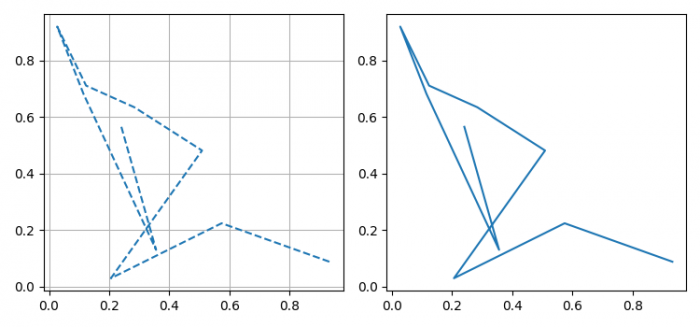# How to set local rcParams or rcParams for one figure in matplotlib?

To set local rcParams or rcParams for one figure in matplotlib, we can take the following steps −

## Steps

• Set the figure size and adjust the padding between and around the subplots.

• Initialize a variable N to store the number of sample data.

• Create x and y data points using numpy.

• Return a context manager for temporarily changing rcParams.

• Add a subplot to the current figure, at index 1.

• Plot the x and y data points, using plot() method.

• Add a subplot to the current figure, at index 2.

• Plot the x and y data points, using plot() method.

• To display the figure, use show() method.

## Example

import pandas as pd
import numpy as np
from matplotlib import pyplot as plt

plt.rcParams["figure.figsize"] = [7.50, 3.50]
plt.rcParams["figure.autolayout"] = True

N = 10

x = np.random.rand(N)
y = np.random.rand(N)

with plt.rc_context({"axes.grid": True, "grid.linewidth": 0.75, "lines.linestyle": 'dashed'}):
plt.subplot(121)
plt.plot(x, y)

plt.subplot(122)
plt.plot(x, y)

plt.show()

## Output

It will produce the following output −